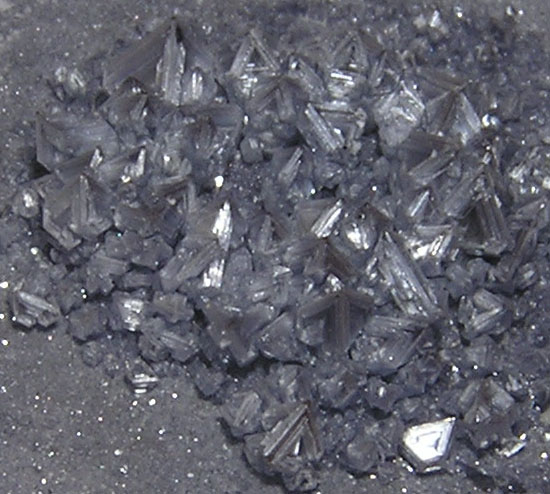## Solution Stoichiometry

#### Learning Objective

• Calculate concentrations of solutions in molarity, molality, mole fraction and percent by mass and volume.

#### Key Points

• Stoichiometry deals with the relative quantities of reactants and products in chemical reactions. It can be used to find the quantities of the products from given reactants in a balanced chemical reaction, as well as percent yield.
• To calculate the quantity of a product, calculate the number of moles for each reactant. Moles of a product are equal to the moles of a limiting reactant in one-to-one reaction stoichiometry. To find product mass, moles must be multiplied by the product’s molecular weight.
• In stoichiometric calculations involving solutions, a given solution’s concentration is often used as a conversion factor.

#### Terms

• stoichiometrythe study and calculation of quantitative (measurable) relationships of the reactants and products in chemical reactions (chemical equations)
• molaritythe concentration of a substance in solution, expressed as the number moles of solute per liter of solution
• molalitythe concentration of a substance in solution, expressed as the number of moles of solute per kilogram of solvent

# Concentration of Solutions

Recall that a solution consists of two components: solute (the dissolved material) and solvent (the liquid in which the solute is dissolved). The amount of solute in a given amount of solution or solvent is known as the concentration. The two most common ways of expressing concentration are molarity and molality.

## Molarity

The molar concentration (M) of a solution is defined as the number of moles of solute (n) per liter of solution (i.e, the volume, Vsolution):

$M=\frac {n}{V_{solution}}$

The units of molarity are mol/L, often abbreviated as M.

For example, the number of moles of NaCl in 0.123L of a 1.00M solution of NaCl can be calculated as follows:

$0.123 \text{ L of solution} \times \frac{1.00 \text{ mole}}{1.00 \text{ L of solution}} = 0.123 \text { moles NaCl}$

## Molality

The molal concentration (m) of a solution is defined as the number of moles of solute (n) per kilogram of solvent (i.e., the mass of the solvent, msolvent):

$m=\frac {n}{m_{solvent}}$

The units of molality are mol/kg, or m.

For example, the number of moles of NaCl dissolved in 0.123kg of H2O (the solvent), in order to make a 1.00m solution of NaCl, can be calculated as follows:

$0.123 \text{ kg of solvent} \times \frac{1.00 \text{ mole}}{1.00 \text{ kg of solvent}} = 0.123 \text { moles NaCl}$

## Reaction Stoichiometry in Solutions

We can perform stoichiometric calculations for aqueous phase reactions just as we can for reactions in solid, liquid, or gas phases. Almost always, we will use the concentrations of the solutions as conversion factors in our calculations.

## Example

• 123 mL of a 1.00 M solution of NaCl is mixed with 72.5 mL of a 2.71 M solution of AgNO3. What is the mass of AgCl(s) formed in the precipitation reaction?

First, we need to write out our balanced reaction equation:

$AgNO_3(aq)+NaCl(aq) \rightarrow AgCl(s) + NaNO_3(aq)$

The next step, as in any calculation involving stoichiometry, is to determine our limiting reactant. We can do this by converting both of our reactants into moles:

$\text{123 mL }NaCl \times \frac{\text{1 L}}{\text{1000 mL}} \times \frac{\text{1.00 mol }NaCl}{\text{1 L}}= \text{0.123 mol }NaCl$

$\text{72.5 mL }AgNO_3 \times \frac{\text{1 L}}{\text{1000 mL}} \times \frac{\text{2.71 mol }AgNO_3}{\text{1 L}}= \text{0.196 mol }AgNO_3$

We can see from our reaction equation that AgNO3 and NaCl react in a 1:1 ratio. Because there are fewer moles of NaCl present in solution, NaCl is our limiting reactant. We can now solve for the mass of AgCl formed:

$\text{123 mL }NaCl \times \frac{\text{1 L}}{\text{1000 mL}} \times \frac{\text{1.00 mol }NaCl}{\text{1 L}} \times \frac {\text{1 mol }AgCl}{\text{1 mol }NaCl} \times \frac {\text{143 g}}{\text{1 mol }AgCl}=\text{17.6 g }AgCl$,

Therefore, 17.6 g AgCl(s) is formed in the reaction.

To sum up: we converted to each reactant’s moles by using the given concentrations as conversion factors, expressing molarity as mol/L; once we found our limiting reactant, we converted through to grams of AgCl formed.Crystals of silver chloride (AgCl)Stoichiometry deals with the relative quantities of reactants and products in chemical reactions. It can be used to find the quantities of the products from given reactants in a balanced chemical reaction, as well as percent yield.